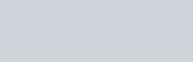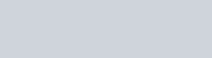Solve graphically each of the following systems of linear equations. Also find the coordinates of the points where the lines meet axis of y.
Question:

Solve graphically each of the following systems of linear equations. Also find the coordinates of the points where the lines meet axis of y.

(i) $2 x-5 y+4=0$

$2 x+y-8=0$

(ii) $3 x+2 y=12$

$5 x-2 y=4$

(iii) $2 x+y-11=0$,

$x-y-1=0$

(iv) $x+2 y-7=0$

$2 x-y-4=0$

(v) $3 x+y-5=0$

$2 x-y-5=0$

(vi) $2 x-y-5=0$,

$x-y-3=0$

Solution:

(i) The given equations are

$2 x-5 y+4=0$…(i)

$2 x+y-8=0$ ..(ii)

The two points satisfying (i) can be listed in a table as,The two points satisfying (ii) can be listed in a table as,Now, graph of equations (i) and (ii) can be drawn as,It is seen that the solution of the given system of equations is given by x = 3, y = 2.

Also, it is observed that the lines (i) and (ii) meet the $y$-axis at the points $\left(0, \frac{4}{5}\right)$ and $(0,8)$ respectively.

(ii) The given equations are

$3 x+2 y=12$….(i)

$5 x-2 y=4$…(ii)

The two points satisfying (i) can be listed in a table as,It is seen that the solution of the given system of equations is given by x = 2, y = 3.

Also, it is observed that the lines (i) and (ii) meet the $y$-axis at the points $(0,6)$ and $(0,-2)$ respectively.

(iii) The given equations are

$2 x+y-11=0$..(i)

$x-y-1=0$..(ii)

The two points satisfying (i) can be listed in a table as,It is seen that the solution of the given system of equations is given by x = 4, y = 3.

Also, it is observed that the lines (i) and (ii) meet the $y$-axis at the points $(0,11)$ and $(0,-1)$ respectively.

(iv) The given equations are

$x+2 y-7=0$…(i)

$2 x-y-4=0$….(ii)

The two points satisfying (i) can be listed in a table as,It is seen that the solution of the given system of equations is given by x = 3, y = 2.

Also, it is observed that the lines (i) and (ii) meet the $v$-axis at the points $(0,3.5)$ and $(0,-4)$ respectivelv.(v) The given equations are

$3 x+y-5=0$…(i)

$2 x-y-5=0$….(ii)

The two points satisfying (i) can be listed in a table as,It is seen that the solution of the given system of equations is given by x = 2, y = −1.

Also, it is observed that the lines (i) and (ii) meet the $y$-axis at the points $(0,5)$ and $(0,-5)$ respectively.

(vi) The given equations are

$2 x-y-5=0$..(i)

$x-y-3=0$..(ii)

The two points satisfying (i) can be listed in a table as,It is seen that the solution of the given system of equations is given by x = 2, y = −1.

Also, it is observed that the lines (i) and (ii) meet the $y$-axis at the points $(0,-3)$ and $(0,-5)$ respectively.